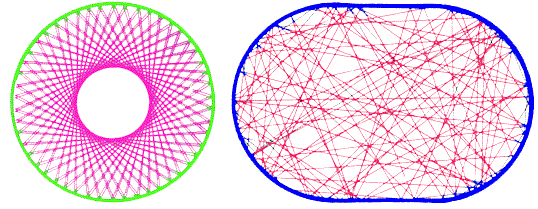Next: Further reading Up: Chaos in Quantum Billiards Previous: Quantum point contacts

# Order versus chaosFigure 4. Trajectories in a circular billiard and a stadium billiard. The motion in the circle is regular or ordered, while in the stadium it is irregular or chaotic.

So much for the holes, now let's turn to the billiard itself. Depending on their shape, billiards come in two types: ordered and chaotic. The trajectories in an ordered and chaotic billiard are contrasted in figure 4. An ordered billiard has a special, highly symmetric shape, such as the circle in figure 4. At each collision with the boundary both the magnitude and the direction of the velocity (relative to the boundary) are the same. The chaotic billiard has no symmetry, so that only the magnitude of the velocity is a constant of the motion. The direction varies in an irregular way from one collision to the next (stadium in figure 4).

In a quantum billiard one can not distinguish order from chaos by inspecting the trajectories. This would require the specification of position and velocity of an electron, which violates the uncertainty principle. So, what happens if we miniaturize the two billiards of figure 4? Is it meaningful to distinguish order from chaos in a quantum billiard? Can one, by measuring the conductance, tell whether the billiard has an ordered or a chaotic shape? These are the outstanding problems in the field of quantum chaos.Next: Further reading Up: Chaos in Quantum Billiards Previous: Quantum point contacts